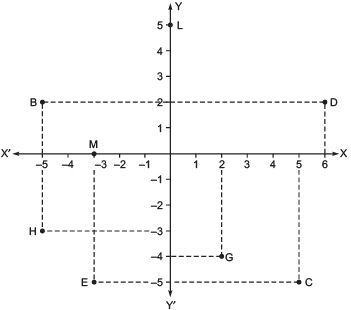# Ex 3.2 NCERT Solutions - Coordinate Geometry Class 9 Notes | EduRev

## Class 9 : Ex 3.2 NCERT Solutions - Coordinate Geometry Class 9 Notes | EduRev

The document Ex 3.2 NCERT Solutions - Coordinate Geometry Class 9 Notes | EduRev is a part of the Class 9 Course Mathematics (Maths) Class 9.
All you need of Class 9 at this link: Class 9

Question 1. Write the answer of each of the following questions:
(i) What is the name of horizontal and the vertical lines drawn to determine the position of any point in the Cartesian plane?
(ii) What is the name of each part of the plane formed by these two lines?
(iii) Write the name of the point where these two lines intersect.
Solution: (i) The horizontal line: x-axis The vertical line: y-axis
(ii) Each part is called: Quadrant
(iii) Origin

Question 2. See the figure given below and write the following:
(i) The co-ordinates of B.
(ii) The co-ordinates of C.
(iii) The point identified by the co-ordinates (–3, –5).
(iv) The point identified by the co-ordinates (2, –4).
(v) The abscissa of the point D.
(vi) The ordinate of the point H.
(vii) The co-ordinates of the point L.
(viii) The co-ordinates of the point M.Solution: From the figure, we have
(i) The co-ordinates of B are (–5, 2).
(ii) The co-ordinates of C are (5, –5).
(iii) The point E is identified by the co-ordinates (–3, –5)
(iv) The point G is identified by the co-ordinates (2, –4).
(v) The abscissa of the point D is 6.
(vi) The ordinate of the point H is –3.
(vii) The co-ordinates of the point L are (0, 5).
(viii) The co-ordinates of the point M are (–3, 0).

Offer running on EduRev: Apply code STAYHOME200 to get INR 200 off on our premium plan EduRev Infinity!

## Mathematics (Maths) Class 9

190 videos|233 docs|82 tests

,

,

,

,

,

,

,

,

,

,

,

,

,

,

,

,

,

,

,

,

,

;